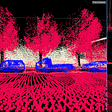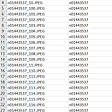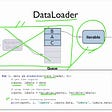# Fitting larger networks into memory.

TLDR; we (OpenAI) release the python/Tensorflow package openai/gradient-checkpointing, that lets you fit 10x larger neural nets into memory at the cost of an additional 20% computation time.

GPU memory is often the limiting factor for modern neural network architectures. Memory requirement to train a neural network increases linearly with both network depth and batch-size. You want to go deeper for standard reasons, but also to increase the batch-size to make use of second order methods like KFAC. Such methods need fewer examples to learn compared to mini-batch SGD.

Today, we release a python/Tensorflow package, openai/gradient-checkpointing, that extends the technique in “Training Deep Nets with Sublinear Memory Cost”, Tianqi Chen et al, to rewrite your TensorFlow model to use less memory. It gives equivalent memory saving for simple feed-forward networks, but it also lets you save memory for general neural networks, such as multi-tower architecture. The package is joint work by Yaroslav Bulatov and Tim Salimans.

Applying it to TensorFlow official CIFAR10 resnet example produces the following memory and execution times for batch size = 1280.

While regular backprop scales linearly, this method scales as square root of depth. The difference is more apparent when we try it out for deeper networks.

Extrapolating memory requirement of standard approach gives 60GB of memory to run this iteration, meanwhile memory saving gradients accomplishes it in 6GB of RAM.

Computation overhead is 1 extra forward pass regardless of depth. In experiments this translated to 20% increase in wall-clock time on GTX1080, and 30% increase in wall-clock time on V100 GPU.

# How memory saving works:

## The pebble game

To understand memory requirements of general computation, computer scientists use the concept of the “pebble game”, introduced in “Complete Register Allocation Problems” by Sethi in 1975. Consider the following computation

You can visualize this computation as a computation graph:

In order to compute each value, you need to have its dependencies loaded into memory. This is represented by placing “pebbles” on the children of the node. Once all children of a node have pebbles on them, the node is ready for execution. Computing its value is represented by placing a pebble on it:

Once the value is not needed anymore, you can remove the pebble from the node and re-use it for later computations:

The goal is to follow the rules of the game to place a pebble on the target node. The number of pebbles needed corresponds to peak memory requirement.

Even for this simple graph, various strategies give different memory requirements. For instance, we can compute the leaves first. That gives following execution strategy, requiring 4 pebbles.

Note that we are computing x4 before it’s needed and keeping its value for a while. We could reduce memory requirement by deferring computation of x4 to a later step.

This gives us strategy requiring 3 pebbles instead of original 4.

A more pronounced difference happens in a computational graph below:

If squares correspond to slow operation like matmul and circles correspond to fast operation like random_uniform, computing things as soon as they are ready will require memory proportional to the length of the chain:

As an alternative, you can defer computations until they are needed, which lets you accommodate arbitrary chain length with 3 units of memory:

Computing values “as soon as possible” (the first strategy) is the default execution strategy used by TensorFlow. You can instruct TensorFlow to compute values “as late as possible” by adding control dependencies. A tool to do this automatically for TensorFlow graphs is linearize.py

Finding the minimum number of pebbles required for general graphs is hard, even approximately, and even if you forbid placing a pebble on any node after it’s been removed (one-shot pebbling). This is shown in “Inapproximability of Treewidth, One-Shot Pebbling, and Related Layout Problems.”

For a simple feed-forward neural network with n layers, gradient computation graph can be pictured below:

See earlier post “Backprop and systolic arrays” for explanation of where this graph comes from.

Using pebbling analogy we can visualize the default strategy to compute backprop in this graph.

At the peak, the algorithm stores all activations, which means O(n) memory requirement for network of depth n. In this case this means 7 units of memory. Unlike the previous example, you can’t save memory by adjusting the order of execution.

You could instead save memory by forgetting nodes as they are consumed and recomputing them later. This strategy, pictured below, needs 4 units of memory to compute the target.

More generally, this “memory-poor” strategy needs O(1) memory but requires O(n²) computation steps.

A compromise is to save some intermediate results. These saved nodes are called “checkpoints” in openai/gradient-checkpointing, and can be either selected automatically or provided manually. For the example above, intermediate strategy could be to use the circled node below as a checkpoint

Using this checkpoint yields a strategy that needs 5 units of memory and has runtime somewhere between memory-poor and default strategies.

For a chain of length n, generalization of this strategy is to place checkpoints every sqrt(n) steps. This is the most memory efficient strategy if you require that any node is computed at most twice. The memory requirement is O(sqrt(n)), and compute requirement is an additional forward pass. This is the strategy we adopt in our package.

Below is the summary of memory and compute requirements of these 3 strategies.

# sqrt(n)

If you monitor memory usage during the evaluation of the gradient in the sqrt(n) strategy, you will see a characteristic zigzag graph like one below.

The first half of the graph corresponds to the first forward pass and saving the initial checkpoints. Spikes represent recomputation of forgotten activations from each checkpoint. Getting graph of within-step memory usage can be done with yaroslavvb/mem_util package.

# Beyond simple feed-forward

What happens if you have a more general architecture? IE, suppose you have a resnet architecture like below:

Note that circular nodes are bad candidates for checkpoints. If you save just the circular nodes, your execution time is as bad as the “memory-poor” strategy for the chain, with O(n²) computation steps:

For deep networks we’d like time complexity to stay at O(n). To achieve this, we could take the square nodes as checkpoint candidates and apply the same sqrt(n) selection strategy on this set.

What makes square nodes special is that knowing the value of each square node removes the need to recompute any nodes before that node. In graph terminology, these nodes are “graph separators” — removing one separates the graph into disjoint subgraphs, “before” subgraph and “after” subgraph.

Graph separators of size 1 are called “articulation points”. This is the class of nodes considered by our package as candidates for checkpoints.

Suppose you have a multi-tower architecture below:

Unlike the previous example, there’s no single node that can serve as a checkpoint candidate. To get any memory saving we must consider nodes in sets of two:

We currently have not automated the selection of such sets of nodes. To get the sqrt(n) benefit in this kind of architecture with our package, you will need to use a manual checkpoint selection strategy. To use separators pictures in the graph above, you would call the package with the following nodes specified as checkpoints: a, b, c, d.

# Ideas for extensions

## Choose strategy automatically

This “sqrt(n)” strategy is useful in practice, but it’s an arbitrary point on the computation/memory trade-off curve, and a better algorithm would minimize computation time subject to memory budget.

Also, this algorithm only applies recomputation during backward pass. Architectures with significant branching like openai/pixel-cnn, run out of memory during forward pass. You can apply similar checkpointing idea to pick nodes to recompute during forward pass.

For instance in the architecture with skip connections we can forget the first node after the middle node is computed, and then compute it again when it’s needed again for the last node.

An ideal algorithm would choose the nodes to recompute in backward pass or the forward pass to give the smallest runtime subject to memory budget.

The default strategy is “memory-rich”: it saves everything that will be needed later, and computes gradient in O(n) memory and O(n) time.

The “memory-poor” strategy is to forget each node as soon as it’s consumed and recompute when it’s needed at a later time. It can compute gradient of network of depth n in O(1) memory and O(n²) time. Example of implementing this strategy in tensorflow is in http://github.com/yaroslavvb/chain_constant_memory/

You can interpolate between “save everything” and “save nothing” above by saving some nodes in an optimal way. This is analyzed in detail in Chapter 12 of “Evaluating derivatives: principles and techniques of algorithmic differentiation”, Griewank A., Walther A, 2nd ed. More recently this technique was applied in Memory-Efficient Backpropagation Through Time.

The idea of the approach is to use dynamic programming to find the most optimal computation schedule.

Building block of DP solution is the algorithm which keeps the first activation in memory and computes the target backprop as fast as possible with memory budget M.

To see how it breaks into smaller parts, suppose the set of nodes checkpointed by this algorithm contained node i. Then this algorithm could be decomposed into parts Left/Right as follows:

Left:

1. Given A0, compute Ai with budget M.

Right:

2. Given Ai, Bn, compute Bi with budget M-M0. M0 is memory cost of first activation which needs to be subtracted since “Left” is keeping the first activation in memory.

Left:

3. Given A0, Bi, Compute B0 with budget M

If the memory budget is too small to save any nodes, then there’s only choice of strategy — the O(n²) memory poor strategy. This is the base case for divide and conquer.

You can go over all choices of ‘i’ as potential nodes to generate initial split, and call the algorithm recursively on individual splits.

TensorFlow lets you you obtain computation times of individual nodes from the same timeline structure as used by the mem_util package, so you can use this information to obtain an optimal schedule based on empirical values of execution times.

## Support general graphs

Dynamic programming algorithm above is formulated for chain graphs, how could it be extended to general computation graphs?

Our checkpoints have to be graph separators in order to reuse recomputation. The algorithm above works on chains because in a chain, every node is a separator. There’s an extension of the dynamic programming approach above to work on trees by using the same divide-and-conquer idea: saving any node in a tree-structured computation graph splits the graph into “before” and “after” parts. The extra complication is that you need to know the order in which to compute the children of each node. If the degree is small enough, a simple exact approach is to try all orders. A heuristic approach is to assume that the most memory-hungry child runs first. An efficient exact strategy was developed in the context of sparse linear systems in “An Application of Generalized Tree Pebbling to Sparse Matrix Factorization” by J. Liu.

For a general computation graph, things are harder. However, note that typical neural network architectures are “pretty close to a tree”. For a multi-tower architecture above, we can merge nodes from parallel paths into sets of nodes, such that resulting graph is a tree.

Now that we have a tree, every node in this representation is a checkpoint candidate and we can apply divide-and-conquer approach over this representation.

In a general graph we use a similar technique known as tree decomposition that works to successively merge sets of nodes until the result is a tree. In tree decomposition, every merged node, also known as bag is a separator of the graph.

For this representation to help with memory saving, merged nodes have to be small. In the worst case you may keep merging nodes and won’t get a tree until everything is merged into a single bag.

This doesn’t happen with neural network examples above which are “pretty close to a tree.” To make this precise, our example graphs have tree decompositions where where largest bag has size k some small k. The number k is the treewidth of a graph.

If the treewidth is small, separators are small, and you can recursively split your search for optimal solution into tractable sub-problems.

Many hard problems on graphs can be solved in polynomial time once tree-width is bounded. This includes the problem of determining the optimal tree decomposition, and the problem of finding optimal pebbling strategy.

More generally such problems are known as fixed-parameter tractable, or FPT, where the fixed parameter is treewidth. Courcelle’s Theorem gives a precise characterization of a set of problems which are FPT for treewidth. A generic algorithm for solving a problem on graphs given its tree decomposition is given in section 5.3.1.2 of Miriam Heinz “Tree-Decomposition: Graph Minor Theory and Algorithmic Implications

# Tree-decompositions of neural nets

Typical architectures have small treewidth. Some examples:

## Feedforward with k-skip connections: k

You can combine these features to get a net with larger, but still bounded, treewidth. IE, if you have a neural network which uses k towers, skip connections in each tower going back k steps and k global statistics, it will have O(k²) treewidth.

Finding optimal tree decomposition for a bounded treewidth graph has a polynomial time algorithm discovered by Bodlaender. Unfortunately this algorithm is not practical, similar to other Galactic Algorithms.

In practice, heuristics find tree-decompositions that are good enough. One particular heuristic is a “min-fill decomposition” which requires a single pass over the graph, and works well for sparsely connected graphs.

I’ve prototyped min-fill heuristic tree decomposition here. The code gives results you see above for toy networks as well as reasonable results for real computational graphs.

IE, for TensorFlow computation graph of resnet:

It produces the following tree structure:

A more advanced algorithm might combine these ideas to find an optimal execution strategy for a general neural network without any input from the user.

# Notes

--

--

--

## More from TensorFlow

TensorFlow is an end-to-end open source platform for machine learning.

## Image Captioning — from theory to implementation## Few-shot Named Entity Recognition in Natural Language Processing## Typesetting neural network diagrams with TeX## How Machine Learning Can Transform The Energy Industry## Machine Learning use case:## A Feast of Sensor Data: Feeding Self-driving Algorithms## This is why ML PoCs don’t graduate to productionasdf

## Part 1: AlphaZero implementation for the game Onitama## Triplet Loss on ImageNet Dataset## Simple Guide to Custom PyTorch Transformations## Custom Dataset with Dataloader in Pytorch